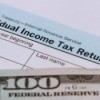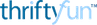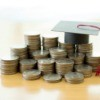# Early Refund Loans are a Bad Deal

Thinking about getting an early refund loan when you have your taxes done? Those early refund loans are a bad idea from a frugal point of view - the effective interest rate on them is sky high.

Example: Let's say you are owed \$500 as a refund and get an early refund loan for \$475 (\$500 - \$25 fee charged by lender). Let's say you would have gotten your refund in 6 weeks anyway so you paid \$25 to have the use of \$475 for 6 weeks. That means your effective interest rate is \$25/\$475 = 5.26%. So you are paying 5.26% in interest every 6 weeks in effect.

If you annualize that rate to see what the annual percentage rate is. First divide 52 into the amount of time in the loan (6/52 = .1154), then divide the interest charged (\$25) by the quotient (.1154) to find what the annual interest charges would be on this loan (\$25/.1154 = \$216.64), finally divide the annual interest charges by the amount of the loan you actually got (\$216.64 / \$475 = 45.61%). This means your effective annual interest rate on the loan is 45.61% which makes these loans a very bad idea for the borrower.

ThriftmeisterCategories
PagesMore
Categories